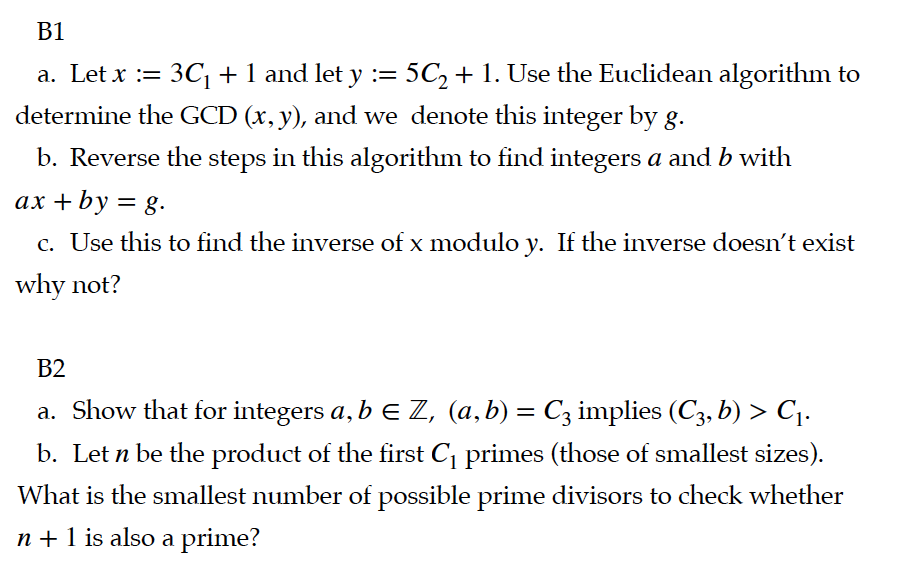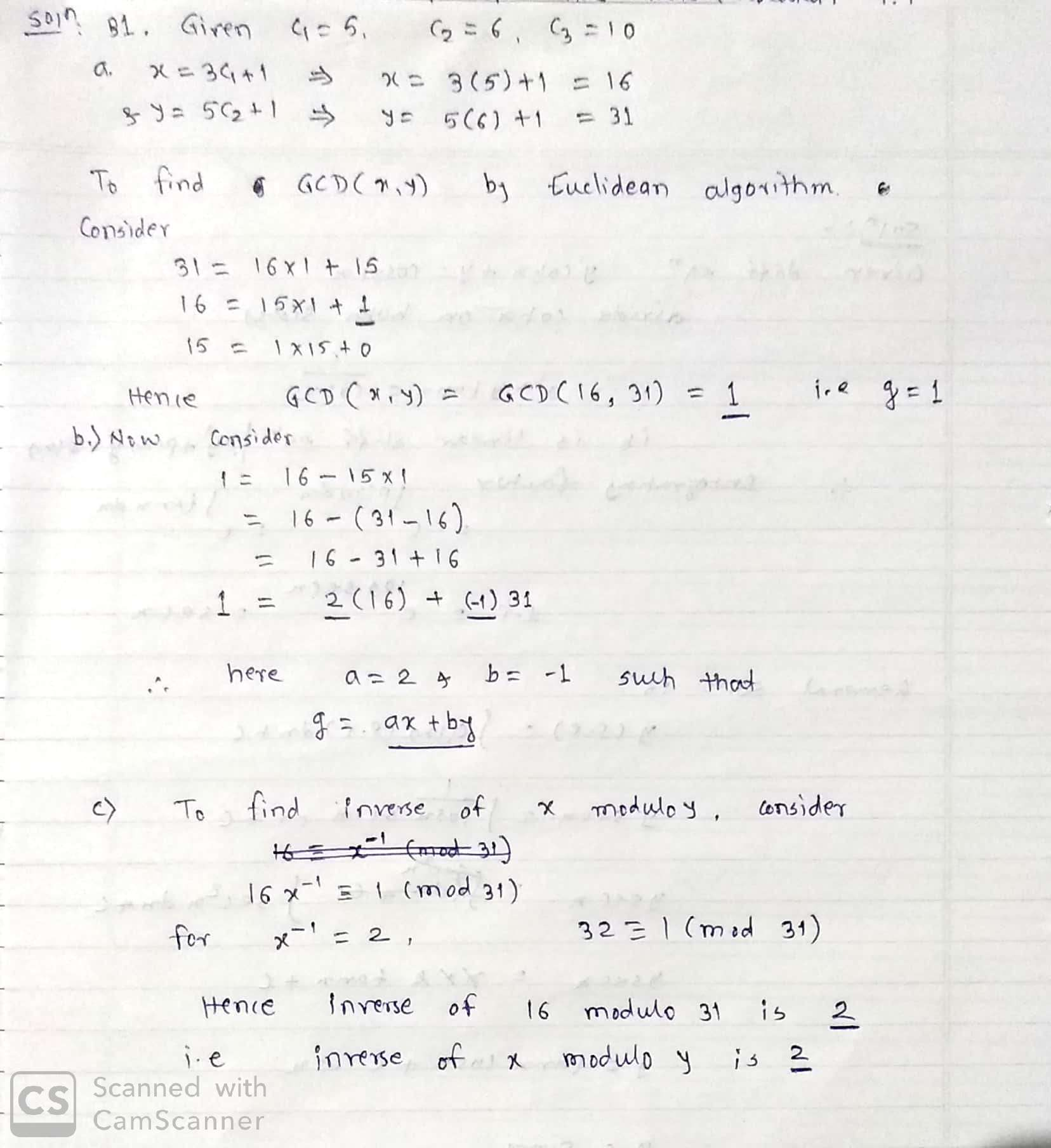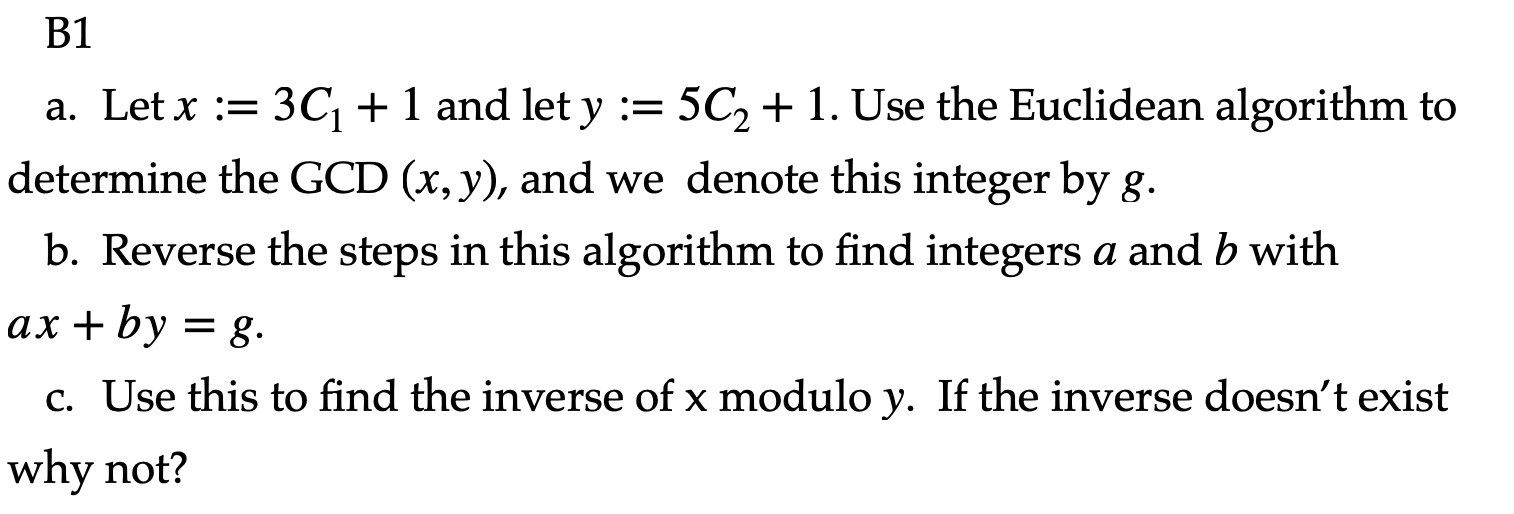Question

# C1= 5 C2= 6 C3= 10 GCD --> Greater Common Divisor B1 a. Let x :=...

C1= 5

C2= 6

C3= 10

GCD --> Greater Common DivisorB1 a. Let x := 3C1 + 1 and let y := 5C2 + 1. Use the Euclidean algorithm to determine the GCD (x, y), and we denote this integer by g. b. Reverse the steps in this algorithm to find integers a and b with ax + by = g. c. Use this to find the inverse of x modulo y. If the inverse doesn't exist why not? B2 a. Show that for integers a, b E Z, (a,b) = Cz implies (C3, b) > C1. b. Let n be the product of the first C primes (those of smallest sizes). What is the smallest number of possible prime divisors to check whether n + 1 is also a prime?#### Earn Coins

Coins can be redeemed for fabulous gifts.

Similar Homework Help Questions
• ### B1 a. Let x := 3C1 + 1 and let y := 5C2 + 1. Use...B1 a. Let x := 3C1 + 1 and let y := 5C2 + 1. Use the Euclidean algorithm to determine the GCD (x, y), and we denote this integer by g. b. Reverse the steps in this algorithm to find integers a and b with ax+by = 8. c. Use this to find the inverse of x modulo y. If the inverse doesn't exist why not?

• ### Write code for RSA encryption package rsa; import java.util.ArrayList; import java.util.Random; import java.util.Scanner; public class RSA...

Write code for RSA encryption package rsa; import java.util.ArrayList; import java.util.Random; import java.util.Scanner; public class RSA {    private BigInteger phi; private BigInteger e; private BigInteger d; private BigInteger num; public static void main(String[] args) {    Scanner keyboard = new Scanner(System.in); System.out.println("Enter the message you would like to encode, using any ASCII characters: "); String input = keyboard.nextLine(); int[] ASCIIvalues = new int[input.length()]; for (int i = 0; i < input.length(); i++) { ASCIIvalues[i] = input.charAt(i); } String ASCIInumbers...

• ### what is the largest prime number you will need to check to find all the prime numbers less than 100 Here is a site that I found searching Google with the key words, "<I>prime numbers</I>

what is the largest prime number you will need to check to find all the prime numbers less than 100Here is a site that I found searching Google with the key words, "prime numbers." http://mathforum.org/dr.math/faq/faq.prime.num.html I hope this helps. Thanks for asking.Finding Primes The Sieve of Eratosthenes Lets find the primes between 1 and 100. Write down the sequence of numbers from 1 to 100. Cross out the 1. Beginning with the 2, strike out every second number beyond the...